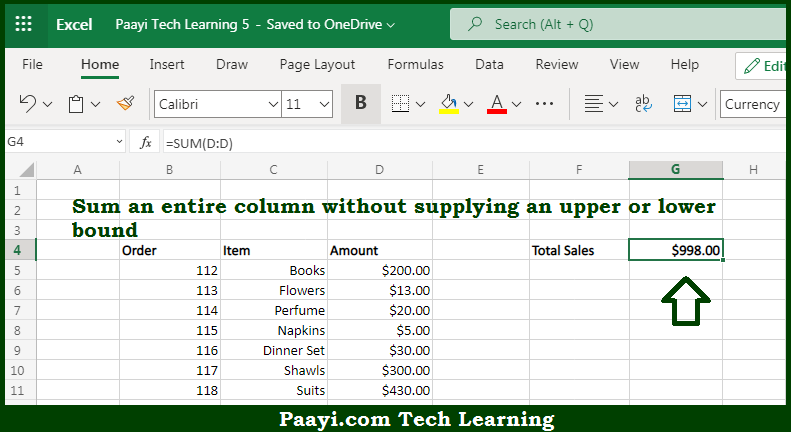# Learn How to SUM Entire Column in Microsoft Excel

Written by | 0 Comments | 510 Views

In this article, you will learn how to COUNT various things in Microsoft Excel using a single/combination(s) of functions. You will also know how to SUM Entire Column and see the generic formula.

SUM Entire Column in Microsoft Excel

The main purpose of this formula is to sum an entire column without supplying an upper or lower bound. Here we will learn how to sum an entire column in the workbook in Microsoft Excel. That implies, with the help of a formula based on the SUM function you can sum an entire column without supplying an upper or lower bound. So, with the help of this formula, you can able to sum an entire column in the workbook in Microsoft Excel.

General Formula to SUM Entire Column

=SUM(A: A)

The Explanation for the SUM Entire ColumnSo we know that with the help of the given formula above you can able to sum an entire column without supplying an upper or lower bound. Here we will learn how to sum an entire column in the workbook in Microsoft Excel. As we know that the full column and row references are an easy way to reference data that have the possibility to change in size. At the same time, you need to be sure that you aren't unintentionally including extra data. For example, if you use =SUM(A: A:) to sum all of column A and column A also includes a date somewhere, this date will be included in the sum. So, with the help of this formula, you can able to sum an entire column without supplying an upper or lower bound.